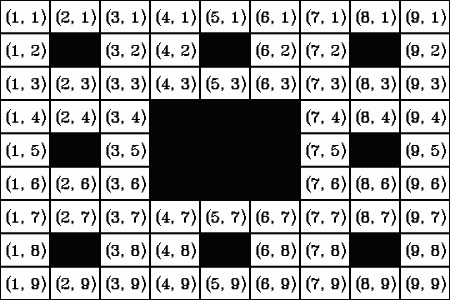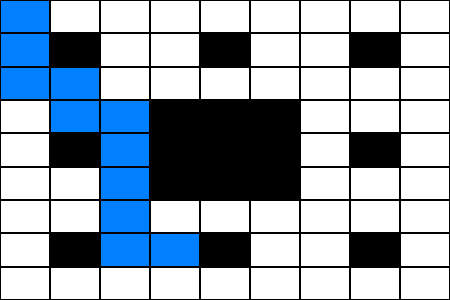# Internet Problem Solving Contest

## Problem H – Here-There

Do you know the game Here-There? I presume you don't. It's a virtual board game, so you should first learn how this virtual board looks like.

The process of making the board is remarkably simple. You start by taking a square with side of length 3N, divide it into nine smaller squares of equal size and remove the central one. Then, you repeat the same divide-and-remove-the-centre process with each of the eight smaller squares over and over (N times in total), until you are left with a grid that consists of many little squares with side length 1 – and of many holes. By the way, the number N is called the degree of the board.

The game itself consists of two steps. First, your opponent chooses two squares on the board, one of them will be "Here" and the other one "There". Your task is to estimate the least number of steps you have to take if you started Here and wanted to get to There. One step consists of moving to another square, which has a common side with the one you're standing on. Obviously, you cannot move over the removed parts of the board. If you guess the number of steps correctly, you get a point.

You would really like to become a master of this game, so you have written down the sizes of the boards and the positions of the Here and There squares from several games in the past. Now, you'd like to find the exact number of steps you need to take to get from Here to There on each of the boards. Each square is described by two numbers between 1 and 3N, the first of them denoting the column and the second one the row the square is in. The square in the upper left corner of the board has coordinates (1, 1), as you can see on the picture below.You can see one of the shortest paths between squares (1, 1) and (4, 8) on the picture, consisting of 10 steps.### Problem specification

You are given several boards and pairs of squares on them and your task is to find the steps-distances between the squares in each pair.

### Input specification

The first line of the input file contains an integer T specifying the number of test cases. Each test case is preceded by a blank line.

Each test case consists of five integers D, Hc, Tc, Hr, Tr, specifying the degree of the board D, the coordinates of Here (Hc, Hr) and the coordinates of There (Tc, Tr).

### Output specification

For each of the test cases, output a single line with one integer – the steps-distance between Here and There.

### Example

Input:
```2

1 1 2 2 1

2 1 1 4 8
```
Output:
```2
10
```

Credits:
Problemsetter(s): g00ber
Contest-related materials: g00ber, Tom